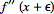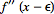# Slight Computation Error? Not sure what I'm doing wrong

• SnakeDoc
would be an example of a point of inflection, because the curve changes its concavity from concave up to concave down.f

## Homework Statement

Water is poured into a container that has a leak. The mass m of the water is given as a function of time t by m = 4.9t.8 - 3.0t + 21, with t ≥ 0, m in grams, and t in seconds.(a) At what time is the water mass greatest, and (b) what is that greatest mass? What is the rate of mass change at (c) t = 2.4 s and (d) t = 5.4 s?
I know that part c and d have to kg/min

## Homework Equations

m=4.9t.8-3.0t+21
d/dm =3.92t-.2-3.0

## The Attempt at a Solution

I got part (a) correct as 3.80911 by finding the points of inflection from the derivatives and then looking at where the equation changes from positive to negative. Part (b) was also easy because all I had to do is plug my answer into the original equation and I got 23.8568361. It's part (c) and (d) that I'm having trouble with.

For part (c) and (d) I know I have to take the derivative so I did and got
3.92t-.2 - 3.0 then I plug in
3.92(2.4)-.2 - 3.0=.2903630437g and 3.92(5.4)^(-.2) - 3.0= -.2022602387g

## Homework Statement

Water is poured into a container that has a leak. The mass m of the water is given as a function of time t by m = 4.9t.8 - 3.0t + 21, with t ≥ 0, m in grams, and t in seconds.(a) At what time is the water mass greatest, and (b) what is that greatest mass? What is the rate of mass change at (c) t = 2.4 s and (d) t = 5.4 s?
I know that part c and d have to kg/min

## Homework Equations

m=4.9t.8-3.0t+21
d/dm =3.92t-.2-3.0

You mean ##\frac{dm}{dt}##.

## The Attempt at a Solution

I got part (a) correct as 3.80911 by finding the points of inflection from the derivatives and then looking at where the equation changes from positive to negative. Part (b) was also easy because all I had to do is plug my answer into the original equation and I got 23.8568361. It's part (c) and (d) that I'm having trouble with.

For part (c) and (d) I know I have to take the derivative so I did and got
3.92t-.2 - 3.0 then I plug in
3.92(2.4)-.2 - 3.0=.2903630437g and 3.92(5.4)^(-.2) - 3.0= -.2022602387g
The numbers are correct for your calculations. I notice that ##m## is given in grams and ##t## in seconds, but for parts c and d you are asking for kg/min. Is the problem correctly stated and, if so, you need to account for the units changes.

I got part (a) correct as 3.80911 by finding the points of inflection
That's not what you mean. You will have found the extrema, by looking for where the first derivative vanishes. A point of inflection is where the second derivative is zero.

That's not what you mean. You will have found the extrema, by looking for where the first derivative vanishes. A point of inflection is where the second derivative is zero.
Not necessarily. A counterexample is y = x1/3. y' is undefined at x = 0, as is y'', but the curvature changes from concave up for x < 0 to concave down for x > 0, and the function is defined at x = 0.

A point of inflection is where the second derivative is zero.
Not necessarily. A counterexample is y = x1/3. y' is undefined at x = 0, as is y'', but the curvature changes from concave up for x < 0 to concave down for x > 0, and the function is defined at x = 0.
Maybe there are different definitions around. E.g. http://en.wikipedia.org/wiki/Inflection_point#Functions_with_discontinuities says a point of inflection has a zero second derivative, but that not all curvature reversals are points of inflection.

Maybe there are different definitions around. E.g. http://en.wikipedia.org/wiki/Inflection_point#Functions_with_discontinuities says a point of inflection has a zero second derivative, but that not all curvature reversals are points of inflection.
I don't see what you're saying in the link you provided. The section titled "Functions with discontinuities" says this:
Some functions change concavity without having points of inflection. Instead, they can change concavity around vertical asymptotes or discontinuities. Take, for example, the function 2x2/(x2 – 1). It is concave when |x| > 1 and convex when |x| < 1. However, it has no points of inflection because 1 and -1 are not in the domain of the function.

One of the calculus books I have (Calculus and Analytic Geometry, 2nd Ed., Abraham Schwartz) has this definition for inflection point (p. 240):
The point P is a point of inflection of curve C if C faces in one direction in a region immediately to the left of P, and in the opposite direction in a region immediately to the right of P.
From this definition, the point (0, 0) on the curve y = x1/3 is an inflection point, even though y'' is not zero at the origin (or even defined there).

I don't see what you're saying in the link you provided.
It says "Instead, they can change concavity around vertical asymptotes". Your ##x^{\frac 13}## exemplifies that. Note the 'instead', implying a vertical asymptote does not count as a point of inflection.
I should also have referenced this further up the same page:
If x is an inflection point for f then the second derivative, f″(x), is equal to zero if it exists,​
Now, that wording is a little odd, but since it is under the heading "A necessary but not sufficient condition", it looks like it means
If x is an inflection point for f then the second derivative, f″(x), exists and is equal to zero​
It certainly should not be taken to mean the second derivative need not exist since for sufficiency it goes on to say
One also needs the lowest-order (above the second) non-zero derivative to be of odd order​

Having said all that, I have to agree that defining inflection points in terms of curvature reversal, (or, equivalently, a change of sign of the second derivative), regardless of whether the sign change is through zero or via infinity, seems a more natural definition.

I don't find it at all surprising that some authorities define it one way, some another.

It says "Instead, they can change concavity around vertical asymptotes". Your ##x^{\frac 13}## exemplifies that. Note the 'instead', implying a vertical asymptote does not count as a point of inflection.
The graph of y = x1/3 has no vertical asymptotes. The tangent to the curve at (0, 0) is vertical, but ##\lim_{x \to 0} x^{1/3}## exists and is equal to 0, so there is no asymptote when x = 0.
haruspex said:
I should also have referenced this further up the same page:
If x is an inflection point for f then the second derivative, f″(x), is equal to zero if it exists,​
The function I gave as an example fits this pattern. We do have an inflection point at (0, 0), but neither f' nor f'' exists.
haruspex said:
Now, that wording is a little odd, but since it is under the heading "A necessary but not sufficient condition", it looks like it means
If x is an inflection point for f then the second derivative, f″(x), exists and is equal to zero​
No, that's not what this is saying. Rather, it's saying that for an inflection point at x = a, if f'' exists, then it must be true that f''(a) = 0. However, we can have an inflection point without f'' existing at the point, as in my example.
haruspex said:
It certainly should not be taken to mean the second derivative need not exist since for sufficiency it goes on to say
One also needs the lowest-order (above the second) non-zero derivative to be of odd order​

Having said all that, I have to agree that defining inflection points in terms of curvature reversal, (or, equivalently, a change of sign of the second derivative), regardless of whether the sign change is through zero or via infinity, seems a more natural definition.

I don't find it at all surprising that some authorities define it one way, some another.

Last edited:
it's saying that for an inflection point at x = a, if f'' exists, then it must be true that f''(a) = 0. However, we can have an inflection point without f'' existing at the point
It doesn't say that. It might be what's meant but it is not what it says. It leaves it quite unclear as to what the test is when the second derivative does not exist. Further, in the sufficiency clause, it requires the existence of some higher order derivative.
You're right that I should not have described your ##x^{\frac 13}## as having a vertical asymptote, I should have said discontinuity, which is the other case dealt with in the same clause. So it still appears that that reference defines it as a curvature reversal but not a point of inflection.

I followed a few more links:

http://mathworld.wolfram.com/InflectionPoint.html
A necessary condition forto be an inflection point is [PLAIN]http://mathworld.wolfram.com/images/equations/InflectionPoint/Inline5.gif. [Broken]
A sufficient condition requiresandto have opposite signs in the neighborhood of [PLAIN]http://mathworld.wolfram.com/images/equations/InflectionPoint/Inline8.gif[/INDENT] [Broken]
(Intriguing: a sufficient condition that fails to imply a necessary condition!)

http://sydney.edu.au/stuserv/documents/maths_learning_centre/thesecondderivative.pdf
A point of inflection occurs at a point where d2y/dx2=0 AND there is a change in concavity of the curve at that point.​

http://clas.sa.ucsb.edu/staff/lee/inflection points.htm
the second derivative must equal zero at that point.
I only found one which supports the other view:
http://www.sosmath.com/calculus/diff/der15/der15.html
[PLAIN]http://www.sosmath.com/calculus/diff/der15/img4.gif[/INDENT] [Broken]

Having researched all that, I conclude that your definition is surely the right one, but most web pages get it wrong.
Thanks for drawing attention to it.​

Last edited by a moderator:
You're right that I should not have described your ##x^{\frac 13}## as having a vertical asymptote, I should have said discontinuity,
I hope that you're referring to the first derivative being is discontinuous, because y = x1/3 is continuous everywhere.
haruspex said:
which is the other case dealt with in the same clause. So it still appears that that reference defines it as a curvature reversal but not a point of inflection.

I found the links you posted to be interesting. The textbooks I used when I was teaching calculus all used definitions similar to the one I posted from Schwartz; i.e., that it wasn't necessary for f'' to be zero in order for an inflection point to be present.